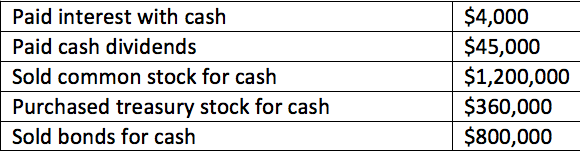# Problem: Consider the following transactions: What was the net cash inflow from financing activities? A. $1,685,000 B.$1,595,000 C. $1,560,000 D.$840,000

###### Problem Details

Consider the following transactions:

What was the net cash inflow from financing activities?

A. $1,685,000 B.$1,595,000

C. $1,560,000 D.$840,000